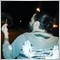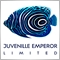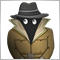# EA difficulty trading on real account.902

I got this EA we wrote, been testing it on demo accounts and works great. but now on a real account it hasn't opened any orders.
Is there any instructions required for the EA to work on a real account?
I have tested it with the Indicators as well over and over.
But for some reason it does not trade on my real account

```#property copyright "Franzel Botha"
#property version   "1.00"
#property strict
input int take_profit = 10;
input int stop_loss = 10;
input double lot_size = 0.1;
input int magic_number = 1234;
input int MA_200 = 200;
input int blue_MA = 25;
input int lime_MA = 50;

double blue,blue1,lime,lime1;
int timeInSeconds;
bool first,second;
int trend,cross;
int changeTime;
int TF,TF1,TF2,TF3,TF4,TF5;
//+------------------------------------------------------------------+
//| Expert initialization function                                   |
//+------------------------------------------------------------------+
int fract_factor = 1;
int OnInit(){
if (Digits == 5 || Digits == 3) fract_factor = 10;
first = false;
second =false;
trend=0;
cross=0;

return(INIT_SUCCEEDED);
}
//+------------------------------------------------------------------+
//| Expert deinitialization function                                 |
//+------------------------------------------------------------------+
void OnDeinit(const int reason)
{
//---

}
//+------------------------------------------------------------------+
//| Expert tick function                                             |
//+------------------------------------------------------------------+
void OnTick()
{
//---
if(!CheckOpenOrders()){

if(!first && change(iBarShift(NULL,0,Time,true),iBarShift(NULL,0,Time,true))==1 && MA200()==1)
{
changeTime=TimeCurrent();
first = true;
trend = 1;
}

if(!first && change(iBarShift(NULL,0,Time,true),iBarShift(NULL,0,Time,true))==-1 && MA200()==-1)
{
changeTime=TimeCurrent();
first = true;
trend = -1;

}

if(first && !second && TimeCurrent()<(changeTime+(30*Period()*60)))
{
if(TimeCurrent()>(changeTime+(Period()*60)) && (change(iBarShift(NULL,0,Time,true),iBarShift(NULL,0,Time,true))==-1||change(iBarShift(NULL,0,Time,true),iBarShift(NULL,0,Time,true))==1))
{
reset();
}

if(MA()==1 && trend==1)
{
second =true;
cross =1;

}
if(MA()==-1 && trend==-1)
{
second =true;
cross =-1;

}

}
if(first && second && TimeCurrent()<(changeTime+(30*Period()*60)))
{
if(TimeCurrent()>(changeTime+(Period()*60)) && (change(iBarShift(NULL,0,Time,true),iBarShift(NULL,0,Time,true))==-1||change(iBarShift(NULL,0,Time,true),iBarShift(NULL,0,Time,true))==1))
{
reset();
}
if(QuantumBreaker::CrossUp(1) && trend==1 && cross==1){
reset();
}
if(QuantumBreaker::CrossDown(1) && trend==-1 && cross==-1){

Order(OP_SELL);
reset();}

}
else if(TimeCurrent()>(changeTime+(30*Period()*60)))
{
reset();
}

}

}
//+------------------------------------------------------------------+

int change(int time,int time1)
{

if((SuperTrend::IsDown(time1)&&SuperTrend::IsUp(time)))
{
return 1;
}
if((SuperTrend::IsDown(time)&&SuperTrend::IsUp(time1)))
return -1;

return 0;
}
bool up(int time)
{
if(SuperTrend::IsUp(time)&&QuantumBreaker::CrossUp(time))return true;
return false;
}
bool down(int time)
{
if(SuperTrend::IsDown(time)&&QuantumBreaker::CrossDown(time))return true;
return false;
}

bool Empty(double value){
return (value == EMPTY_VALUE || value == 0);
}

double GetIndicator(int mode,int shift){
return iCustom(Symbol(),Period(),"",mode,shift);
}

class QuantumBreaker{

public:

static double Get(int mode, int shift){
return iCustom(Symbol(), Period(), "Quantum Breaker V1.0", mode, shift);
}

static bool CrossUp(int shift){
if(QuantumBreaker::Get(4,shift) < QuantumBreaker::Get(3,shift)
&& QuantumBreaker::Get(4,shift+1) > QuantumBreaker::Get(3,shift+1)
)
return true;
return false;
}

static bool CrossDown(int shift){
if(QuantumBreaker::Get(4,shift) > QuantumBreaker::Get(3,shift)
&& QuantumBreaker::Get(4,shift+1) < QuantumBreaker::Get(3,shift+1)
)
return true;
return false;
}

};

bool CheckOpenOrders(void){
//We need to scan all the open and pending orders to see if there is there is any
//OrdersTotal return the total number of market and pending orders
//What we do is scan all orders and check if they are of the same symbol of the one where the EA is running
for( int i = 0 ; i < OrdersTotal() ; i++ ) {
//We select the order of index i selecting by position and from the pool of market/pending trades
//If the pair of the order (OrderSymbol() is equal to the pair where the EA is running (Symbol()) then return true
if( OrderMagicNumber()==magic_number)return(true);}
//If the loop finishes it mean there were no open orders for that pair
return(false);
}

int Order(int order_type){

double tp = (take_profit == 0) ? 0 : (order_type == OP_BUY) ? Ask + take_profit * Pip() : Bid - take_profit * Pip();
double sl = (stop_loss == 0) ? 0 : (order_type == OP_BUY) ? Ask - stop_loss * Pip() : Bid + stop_loss * Pip();
double lot = lot_size;
lot = NormalizeDouble(lot,2);
return OrderSend(Symbol(),order_type,lot,price,10,sl,tp,StringConcatenate(magic_number+" "+Symbol()),magic_number,0,clrNONE);
}

double Pip(){
return Point * 10;
}

class SuperTrend{

public:

static double Get(int mode, int shift){
return iCustom(Symbol(), Period(), "SuperTrend MT4 Indicator",mode,shift);
}

static bool IsUp(int shift){
if(SuperTrend::Get(0,shift) != 0 && !Empty(SuperTrend::Get(0,shift)))
return true;
return false;
}

static bool IsDown(int shift){
if(SuperTrend::Get(1,shift) != 0 && !Empty(SuperTrend::Get(1,shift)))
return true;
return false;
}
};

datetime CloseTimeOfOrder(int magic)
{

int tNumber=0;
double tProfit=0;

{
OrderSelect(x,SELECT_BY_POS,MODE_HISTORY);
if(OrderMagicNumber()==magic)
{

{
OrderSelect(xx,SELECT_BY_POS,MODE_HISTORY);
if(OrderMagicNumber()==magic)
{
{
//tNumber=OrderTicket();
//tProfit=OrderProfit();
// string oType=OrderType();
}
}
}

}
}

}

int MA (void)
{
blue=iMA(Symbol(),0,blue_MA,0,MODE_SMA,PRICE_CLOSE,1);
lime=iMA(Symbol(),0,lime_MA,0,MODE_SMA,PRICE_CLOSE,1);
blue1=iMA(Symbol(),0,blue_MA,0,MODE_SMA,PRICE_CLOSE,2);
lime1=iMA(Symbol(),0,lime_MA,0,MODE_SMA,PRICE_CLOSE,2);

if(blue1<lime1 && blue>lime)
{
return 1;
}
if(blue1>lime1 && blue<lime)
{
return -1;
}
return 0;

}

void reset (void)
{
first = false;
second =false;
trend=0;
cross=0;

}
int MA200 (void)
{
blue=iMA(Symbol(),0,MA_200,0,MODE_SMA,PRICE_CLOSE,1);
//lime=iMA(Symbol(),0,lime_MA,0,MODE_SMA,PRICE_CLOSE,1);
//blue1=iMA(Symbol(),0,MA,0,MODE_SMA,PRICE_CLOSE,2);
//lime1=iMA(Symbol(),0,lime_MA,0,MODE_SMA,PRICE_CLOSE,2);

if(Close>blue && Close<blue)
{
return 1;
}
if(Close<blue && Close>blue)
{
return -1;
}
return 0;

}```20208

Franzel Botha:
I got this EA we wrote

been testing it on demo accounts and works great.

But for some reason it does not trade on my real account

1. Who's we? Why aren't you asking them?
2. So you didn't find all the bugs, no supprise there?
3. We are not going to debug your hundreds of lines of code. Use the debugger or print out your variables, including _LastError and find out why.

4. ```      OrderSelect( i, SELECT_BY_POS, MODE_TRADES );

return OrderSend(Symbol(),order_type,lot,price,10,sl,tp,StringConcatenate(magic_number+" "+Symbol()),magic_number,0,clrNONE);
```
Check your return codes for errors, report them and you would know why. Don't just silence the compiler, it is trying to help you.
What are Function return values ? How do I use them ? - MQL4 and MetaTrader 4 - MQL4 programming forum
Common Errors in MQL4 Programs and How to Avoid Them - MQL4 Articles
Only those functions that return a value (e.g. iClose, MarketInfo, etc.) must you call ResetLastError before in order to check after.902

whroeder1:
1. Who's we? Why aren't you asking them?
2. So you didn't find all the bugs, no supprise there?
3. We are not going to debug your hundreds of lines of code. Use the debugger or print out your variables, including _LastError and find out why.

No need to be rude.

1. a family member and I did the project together and we got stuck.

2. there are no bugs when the indicators are loaded and the EA works %100 on a demo account. just refuses to trade on real account.

3. I am not asking for anyone to debug the Coding. I want to know if I need to insert a specific command so that it will trade on a real account.Moderator
10225

Watch your language, especially with a member like whroeder1, who helps others whenever he can.

Thats better, I see you edited your post.902

Eleni Anna Branou:

Watch your language, especially with a member like whroeder1, who helps others whenever he can.

sorry but he was rude.
I just asked a simple question and he bombarded me.Moderator
10225

Franzel Botha:

sorry but he was rude.
I just asked a simple question and he bombarded me.

He usually does so (bombard), but only in order to help.902

Eleni Anna Branou:

He usually does so (bombard), but only in order to help.

could have done it in a more presentable way though. but its ok. I apologize.7864

One of the inaccuracies.

`OrderSelect( i, SELECT_BY_POS, MODE_TRADES );`

It will be more correct

`if( !OrderSelect( i, SELECT_BY_POS, MODE_TRADES ) ) continue;`
And so all OrderSelect902

Konstantin Nikitin:

One of the inaccuracies.

It will be more correct

great. thank you for the indication902

Konstantin Nikitin:

One of the inaccuracies.

It will be more correct

And so all OrderSelect

I cleaned up the code and did as you said Thank you so much for the direction. I am only sitting with these two warnings now.

you maybe got any idea what it could be?

Stating the first one at the first magic number
implicit conversion from 'number' to 'string' Super MA Quantum v7 MA200.mq4 208 78

Stating the second one
possible use of uninitialized variable 'lastTradeTime' Super MA Quantum v7 MA200.mq4 270 8

```return OrderSend(Symbol(),order_type,lot,price,10,sl,tp,StringConcatenate(magic_number+" "+Symbol()),magic_number,0,clrNONE);`return lastTradeTime=0;  `